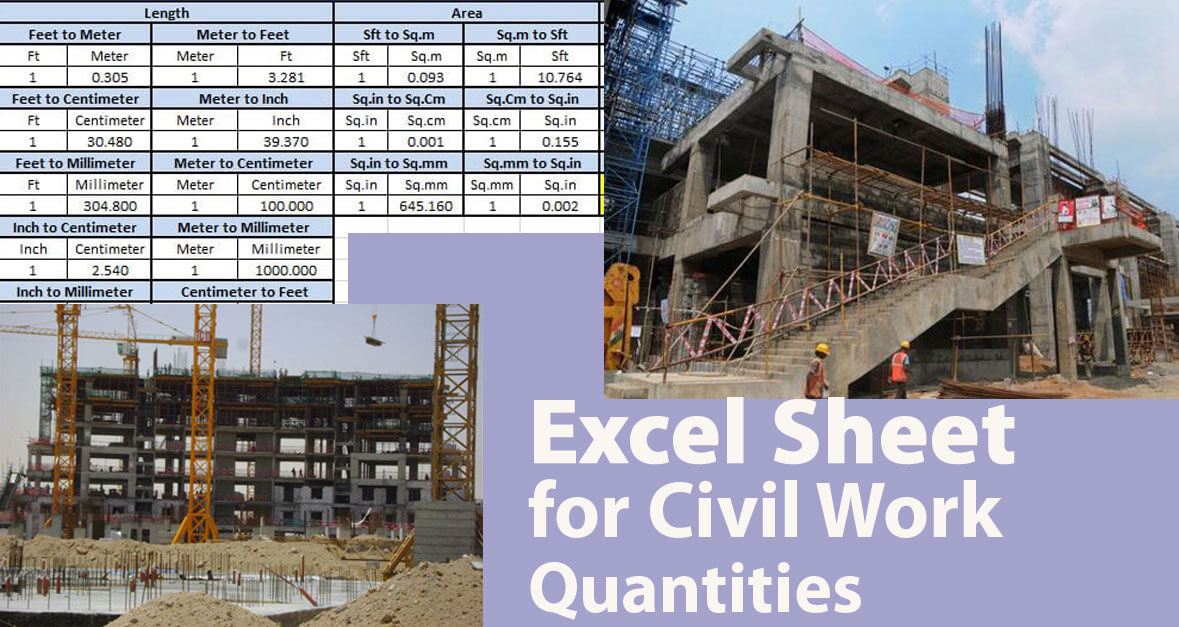# Excel Sheet for Civil Work Quantities

The question often arises on why you would like excel sheet for civil work quantities?

The Excel sheet with all the formula arranged is extremely useful for calculation, as what you've got to try to to is simply put within the values and obtain the result directly and quickly.

Advantages of Excel Sheet for Civil Work Quantities

Stated below are a number of the benefits of using excel sheet for civil work quantities:

1. You can use it to calculate Brick Masonry Work.
• Masonry Volume
• Number of Bricks
• Ratio
• Cement
• Sand
2. Concrete Work can also be calculated here
• Concrete Work for Ft
• Concrete Work for Meter
• Steel in R.C.C for Meter
• Steel in R.C.C for Ft
3. You can also use it to calculate Plaster Work and Quantities such as
• Plaster Work for Ft
• Plaster Work for Meter
4. The excel sheet can also be used to calculate no of the tiles floor area of tiles
• Tile Work for Ft
• Tile Work for Meter
5. Since the converter is attached in the excel sheet, you can use it to convert any values from mks to cgs or mks to fps.
Contents of the Excel Sheet

The excel sheet contains for possible formula which are required for construction site. Stated after are some of the points which the excel sheet covers.

• Brick Masonry Work
• Block Masonry Work
• Concrete Work
• Plaster Work
• Tile Work
• Converter
• Meter to feet
• Feet to Meter
• Area
• Sft to Sq.m
• Sq.m to Sft
• Feet to Centimeter
• Inch to Centimeter
• Inch to Millimeter
• Sooter to Millimeter
• Mile to Km
• Km to Mile
• Mile to Meter
• Mile to Feet
• Km to Meter
• Km to Feet
• Sq.in to Sq.mm
• Ton to Kg
• Kg to Ton
• Kg to Lbs

By following this link, you will be able to get access to this excel sheet, where you can get an idea regarding how to design an excel sheet and what it must contain: drive.google.com